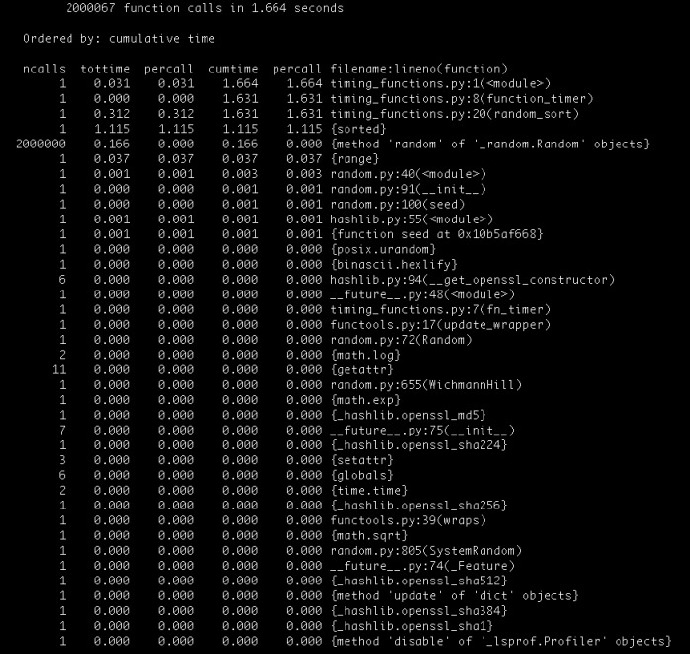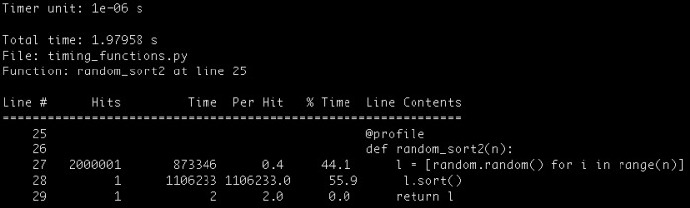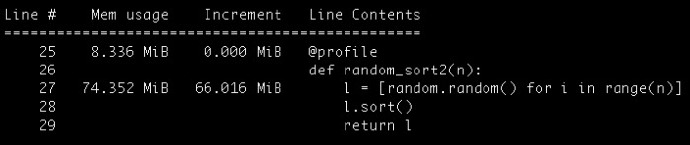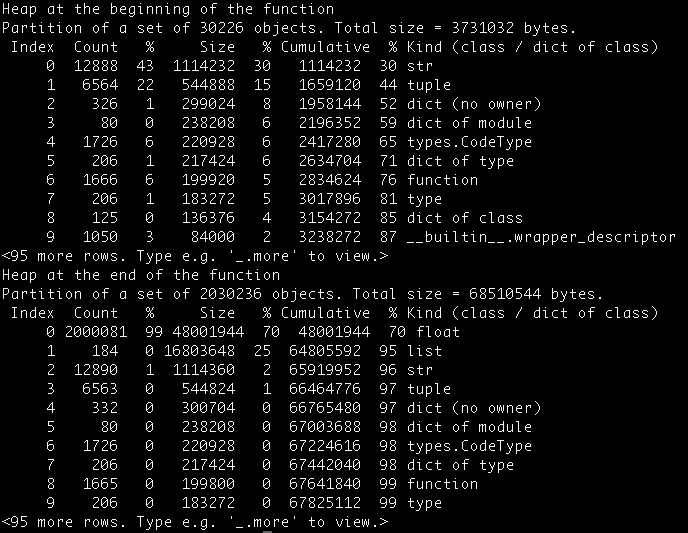• 10种检测Python程序运行时间、CPU和内存占用的方法
• 2017年12月24日
• 网络收集

1. 使用装饰器来衡量函数执行时间

```import time
from functools import wraps
def fn_timer(function):
@wraps(function)
def function_timer(*args, **kwargs):
t0 = time.time()
result = function(*args, **kwargs)
t1 = time.time()
print ("Total time running %s: %s seconds" %
(function.func_name, str(t1-t0))
)
return result
return function_timer
```

```@fn_timer
def myfunction(...):
...
```

```@fn_timer
def random_sort(n):
return sorted([random.random() for i in range(n)])
if __name__ == "__main__":
random_sort(2000000)
```

```Total time running random_sort: 1.41124916077 seconds
```

2. 使用timeit模块

```python -m timeit -n 4 -r 5 -s "import timing_functions" "timing_functions.random_sort(2000000)"
```

```4 loops, best of 5: 2.08 sec per loop
```

3. 使用Unix系统中的time命令

```\$ time -p python timing_functions.py
```

```Total time running random_sort: 1.3931210041 seconds
real 1.49
user 1.40
sys 0.08
```

•     real表示的是执行脚本的总时间
•     user表示的是执行脚本消耗的CPU时间。
•     sys表示的是执行内核函数消耗的时间。

4. 使用cProfile模块

```\$ python -m cProfile -s cumulative timing_functions.py
```5. 使用line_profiler模块

line_profiler模块可以给出执行每行代码所需占用的CPU时间。

```\$ pip install line_profiler
```

```@profile
def random_sort2(n):
l = [random.random() for i in range(n)]
l.sort()
return l
if __name__ == "__main__":
random_sort2(2000000)
```

```\$ kernprof -l -v timing_functions.py
```6. 使用memory_profiler模块

memory_profiler模块用来基于逐行测量代码的内存使用。使用这个模块会让代码运行的更慢。

``` pip install memory_profiler
```

```\$ pip install psutil
```

```\$ python -m memory_profiler timing_functions.py
```7. 使用guppy包

```\$ pip install guppy
```

```from guppy import hpy
def random_sort3(n):
hp = hpy()
print "Heap at the beginning of the functionn", hp.heap()
l = [random.random() for i in range(n)]
l.sort()
print "Heap at the end of the functionn", hp.heap()
return l
if __name__ == "__main__":
random_sort3(2000000)
```

```\$ python timing_functions.py
```